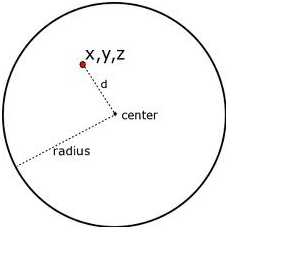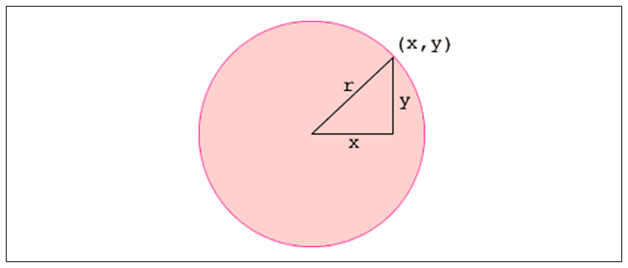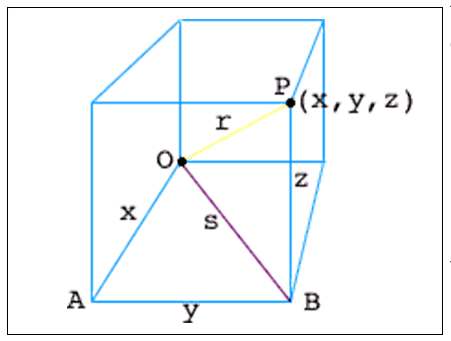# Equation of Sphere

A sphere is defined as a completely round geometrical object in a three-dimensional space just like a round ball. To be geometrical, a sphere is a set of points that are equidistant from a point in space. The distance between the outer point and centre of the sphere is called the radius, denoted by r and the maximum straight distance between any two sides of the sphere through the centre is known as the diameter, denoted by d.A hemisphere is exactly half of a sphere which can only be obtained when a sphere is split from the middle. The biggest circle of a sphere is a circle that has the same centre and radius of a sphere. A great circle of the sphere is a circle that has the same radius and centre as the sphere itself. In this article, let us discuss how to derive the equation of a sphere along with the surface area and the volume of the sphere in detail.

## How to Derive the Equation of a Sphere?

The equation of a circle of radius r is given by:

x2 + y2 = r2

You can relate it to the algebraic method of starting the Pythagoras theorem.The point (x,y) lies on the circle only when the right triangle has sides of length |x| and |y| and hypotenuse of length r, which can be written as:

x2 + y2 = r2

Pythagoras theorem can be used twice for the equation of a sphere. In the below figure, O is the origin and P(x,y,z) is a point in three-space. P is on the sphere with radius r only when the distance from O to P is r.Since OAB is a right angle triangle, x2 + y2 = s2. The triangle OBP is another right triangle and therefore, s2 + z2 = r2. Hence, the distance between O and P can be expressed by:

x2 + y2 + z2 = |OP|2

Hence, we can conclude that (x,y,z) lies on the sphere with radius r only if,

x2 + y2 + z2 = r2

which is called the equation of a sphere.

If (a, b, c) is the centre of the sphere, r represents the radius, and x, y, and z are the coordinates of the points on the surface of the sphere, then the general equation of a sphere is (x – a)² + (y – b)² + (z – c)² = r²

## Volume of a Sphere Equation

The formula to calculate the volume of a sphere is given by the equation:

The volume of the sphere =

$$\begin{array}{l}\frac{4}{3} \pi r^{3}\end{array}$$

Where r is the radius of the sphere.

## Surface Area of a Sphere Equation

The formula to calculate the surface area of the sphere is given by:

The Surface area of the sphere=

$$\begin{array}{l} 4 \pi r^{2}\end{array}$$
square units.

### Equation of a Sphere Example

Example:

Write the equation of the sphere in the standard form where the centre and radius of the sphere are given as (11, 8, -5) and 5 cm respectively.]

Solution:

Given: Centre  = (11, 8, -5)  = (a, b, c)

We know that the equation of the sphere in the standard form is written as:

(x-a)2 + (y-b)2 + (z-c)2 = r2

Now, substitute the given values in the above form, we get:

(x-11)2 + (y-8)2 + (z -(-5))2 =52

(x-11)2 + (y-8)2 + (z +5)2 = 25

Thus, the equation of the sphere is (x-11)2 + (y-8)2 + (z +5)2 = 25

To know more about the properties of spheres and along with operations and problems, you can visit BYJU’S – The Learning App.

Test your Knowledge on Equation Of Sphere